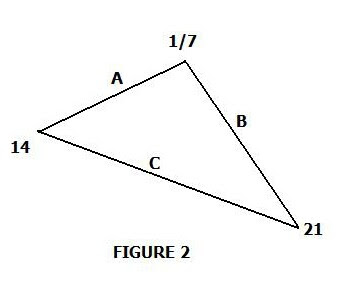## Friday, March 13, 2020

### Revisiting The Math Of p-adicsWhat are the p-adics? They're actually a specialized class of numbers first uncovered by Kurt Densel in the late 1800s. A key aspect is their absolute value, which depends on the prime number on which any p-adic is based. The p then means the particular prime. Given primes of 2, 3, 5, 7 - for example, one can find 2-adic, 3-adic, 5-adic and 7-adic absolute values which are always computed by taking the reciprocal of the highest multiple of p which divides into any given natural number, N.

If N has no multiples of p, then the absolute value is simply 1. If we are looking at a p-adic absolute value of zero, the result is always zero. (E.g.  p = 0)

Let's look at some examples before examining more elaborate applications. Consider the 3-adic versions of: 7, 5 and 1/3. What will the 3-adic absolute values be? Compute each in turn:

 3  = 1 (since there are NO multiples of 3 to form the number N = 7)

 3  = 1 (for the same reason, thus:  3 =  )

[1/3 ] 3 =   3 /   3 = 1/ (1/3) = 3

Since the reciprocal of 3 is 1/3 which we then divide into the numerator 1.

 2

[1/ 6 ] 2

[1/ 8 ] 3

[24 / 25 ] 2

The first is pretty easy, since:   =   [2 x 2] 2 = 1 /4

The next isn't  terribly difficult either:

[1/ 6 ]  =  [1 ] 2   /   [3 x 2  ] 2 = 1/(1/2) = 2

and: [18 ] 3   = [1 ] 3   / [8 ] 3  = 1/  1 = 1

Note in this case, since the denominator (8) has no 3-factors, it must be that  [8 ] 3 = 1

Lastly:

[24 / 25 ]  = [3 x 2 x 2 x 2] 2     2 = (1/8)/ 1 = 1/8

(Again, 25 has no multiples of 2 which can compose it, so [25 ] 2 = 1)

Even more intriguing are the spatial relations and differences, divergences between normal space and p-adic space. Consider the triangle (scalene) shown in Fig. 1 and the linear dimensions (absolute values) of its respective sides. We find: A = 4 ([4 - 0]); B = 6 ([10 - 4]); and C = 10 ([0 - 10]). Now compute the sides using 2-adic absolute values (I will assume the reader can perform the end computations based on the previous examples):

for A: [0 - 4] 2 = 1 / 4

for B: [4 - 10] 2 = [1/4 - 1/2] = 1/4

for C: [0 - 102 =  2 = 1/2

Amazingly, in the p-adic context we find the counter-intuitive result that side A equals side B. In other words, in this context, the triangle is found to be isosceles! A general rubric is that for any such computations of the p-adic absolute values contingent on a given triangle's sides - there will always be found an isosceles triangle - irrespective of how the triangle appears in normal space.

Even more bizarre results await when we examine apparently infinite series in the p-adic context. Thus, a series that first appears to go on to an infinitely large extent may be found much more different when p-adics enter. Consider the series given by a sum:

S = 1 + 5 + (5) 2  + (5) 3  + (5) 4  + (5) 5  + (5)  6  + .........

To treat S p-adically, multiply both sides by 5, then place the result under the original S and subtract:

->

S = 1 + 5 +  (5) 2  + (5) 3  + (5) 4  + (5) 5  + (5)  6  + .+ .........

5 S = 5   +  (5) 2    + (5) 3   + (5) 4  + (5) 5 + (5)  6  + .+ .........
______________________________________________

S - 5S = 1 (all other terms above and below cancel out!)

So: -4S = 1 and S = -1/4

In other words, the sum S is less than 1 in the p-adic form - totally counter-intuitive! We see that evidently the notion or concept of "closeness" emerges quite differently - certainly if we can turn an "infinite" (apparently) sum into one yielding a result less than one!

Problems for the Math whiz:

1- For the triangle in Fig. 2, use 7-adic absolute values applied to the sides of the triangle, thereby compute: A, B and C and show it is isosceles.

2- Find the value of the sum S for: S = 1 + 7 +  (7) 2    + (7) 3   + (7) 4  + (7) 5  + .......

3- Using (2) as written, "invent" a new irrational number based on the p-adic form.